# Five Indicators To Build A Trend Following Strategy

Trend following strategies are strategies where you simply ride the trend, i.e. buy when the price is going up and sell when the price starts going down. In this strategy, one does not aim to forecast or predict, but simply keep an eye on the market for any emerging trends.

#### How does a trend emerge though?

We have all heard of things going viral, thanks to the power of the internet. It’s the same concept albeit, with a different goal. In the financial world too, there is FOMO (Fear Of Missing Out), although here, the reason is a general desire to be on the winning side. Emotions drive people.

While we use algorithmic trading i.e quantitative trading strategies to curb sentimental trading, the same can also be used to utilize and monetize sentiments.

#### How is a trend following strategy implemented?

Trend Following strategies aims to leverage market scenarios profitably. Reason being the high amount of risk and equally high amount of benefits attached to the same. Opinions of influencers and market leaders formulate a general perception and create an on-going buzz around matters of general interest. Elaborating further on this specific investment strategy – the functionality of the trend following strategy is based on the technical analysis of the market data. Since the objective is to measure the intangible aspects pertaining to trading, the first and foremost task is to identify the parameters that govern the situation.

No single indicator can predict a secure way to buy or sell a security. However, there are a few famous ones which are employed frequently to gain an analytical perspective and logical decision-making. The following are the best trading indicators which will help create a trend following strategy### Moving Averages

Moving Averages indicator is a widely used technical indicator that is used to arrive at a decision that is not based on one or two episodes of price fluctuations. A set of historical data can be employed to observe the price fluctuations of the stock for a predetermined period of time. The same assists in depicting the general direction of the trend flow. This technique is used for generating support and building resilience for future outcomes.

#### How to use Moving averages in trend following strategies:

Moving averages provide a clear idea of whether to take a long or short position on the stock. If the stock depicts a negative trend ie the price is below the moving average, take a short position (sell) on the stock. On the other hand, if the stock price is above the simple moving average, one has to take a long position (buy) on the stock because there is an expectancy of the stock price rising further.

#### Plotting Moving averages in python for trend following strategies:

Before we plot the moving averages, we will first define a time period and choose a company stock so that we can analyse it. For this article, let us keep the range as 1st January 2017 to 1st January 2018, and the company details to be used is Tesla (TSLA).

Thus, importing the relevant library and defining the period in python is given in the following code:

```from iexfinance import get_historical_data
from datetime import datetime
import pandas as pd

start = datetime(2017,1,1)
end = datetime(2018,1,1)
data = get_historical_data('TSLA', start =start, end=end, output_format='pandas')

data.index= pd.to_datetime(data.index)

Now, we plot the SMA and EMA of the company, using the following python code:

```# Import libraries
import talib as ta
import matplotlib.pyplot as plt
import seaborn as sns
plt.style.use('bmh')
#Simple Moving Average
data['SMA'] = ta.SMA(data.close, timeperiod = 20)
# Exponential Moving Average
data['EMA'] = ta.EMA(data.close, timeperiod = 20)
# Plot
data[['close','SMA','EMA']].plot(figsize=(10,5))
plt.show()```

The graph plotted is shown below: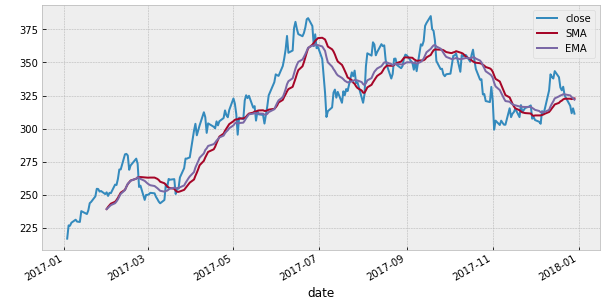### Bollinger Bands

Bollinger band indicators are signals plotted on a singular line which represent the price fluctuations for a particular stock. They consist of three lines, Upper Bollinger band, Middle band, Lower Bollinger band. The upper and lower Bollinger bands are plotted two standard deviations away from the mean average. The two signals or the bands are plotted to measure the volatility of the price fluctuations.

#### How to use Bollinger bands in trend following strategies:

When markets become more volatile, the distance between the signals increases or in short the bandwidth widens and the reverse for low volatility. Higher the volatility, higher the cue for quitting the trade.

The reason the Bollinger bands are plotted two standard deviations away from the mean average is to make sure that the distance between the two bands comprises more than 80% of the price action, thus making any price above or below the bands highly significant.

#### Plotting Bollinger Bands in python for trend following strategies:

The python code is given below:

```# Bollinger Bands
data['upper_band'], data['middle_band'], data['lower_band'] = ta.BBANDS(data.close, timeperiod =20)
# Plot
data[['close','upper_band','middle_band','lower_band']].plot(figsize=(10,5))
plt.show()```

The graph plotted is shown below: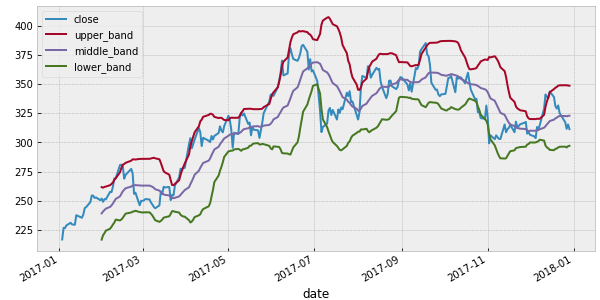### MACD (Moving Average Convergence Divergence)

The Moving Average Convergence Divergence indicator (MACD) is a comparative analysis of two moving averages for two different datasets. Depending on the bandwidth of the time series, you can assess the price fluctuations for two different stretches of time. Say one for a span of a month and another for 200 days. Comparison of the moving average for these two data sets is done based on three main observations viz convergence, divergence and dramatic rise.

#### How to use MACD in trend following strategies:

If the price fluctuations for one data set is less than the moving average while for the other data the fluctuations are above the moving average, it is wiser to take a short position on the stock because the price variation is not stable.

#### Plotting MACD in python for trend following strategies:

The python code is given below:

```# MACD
data['macd'], data['macdsignal'], data['macdhist'] = ta.MACD(data.close, fastperiod=12, slowperiod=26, signalperiod=9)
data[['macd','macdsignal']].plot(figsize=(10,5))

plt.show()```

The graph plotted is shown below: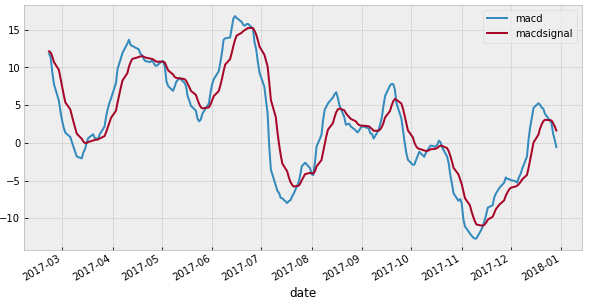### RSI (Relative Strength Index)

The relative strength index ie RSI indicator is calculated using the following formula:

RSI = 100 – 100 / (1 + RS)

where RS = Average gain of up periods during the specified time frame / Average loss of down periods during the specified timeframe.

RSI is used to measure speed and change of the price fluctuations. This indicator provides an idea of the security’s recent performance in the stock market. It measures the strength of the stock in the range of zero to a hundred.

#### How to use RSI in trend following strategies:

A stock is considered overbought over the range of 70 and oversold below 30. You can modify your trading strategy accordingly.

#### Plotting RSI in python for trend following strategies:

The python code is given below:

```# RSI
data['RSI'] = ta.RSI(data.close, timeperiod=14)
# Plotting RSI
fig,ax = plt.subplots(figsize=(10,5))
ax.plot(data.index, data.RSI, label='RSI')
ax.fill_between(data.index, y1=30, y2=70, color = 'lightcoral', alpha='0.3')
ax.set_xlabel('Date')
ax.set_ylabel('RSI')
plt.show()```

The graph plotted is shown below: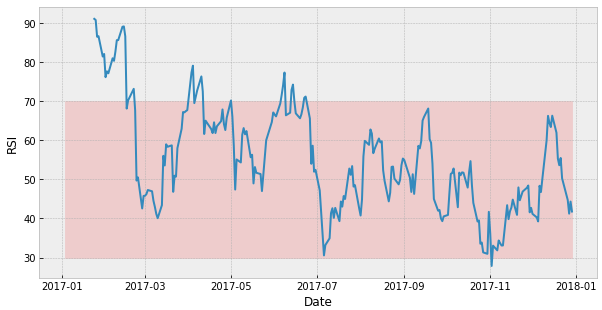### OBV (On Balance Volume)

OBV Indicator is a momentum based indicator which measures volume flow to gauge the direction of the trend. Volume and price rise are directly proportional. A rising price is depicted by a rising OBV and a falling OBV stands for a falling price. If OBV depicts a rise in the same pattern as the prices this is a positive indicator. While a contrast with the pattern depicts a negative indicator.

#### How to use OBV in trend following strategies:

OBV is used as a confirmation tool with regards to price trends. If the OBV increases with respect to the increasing price trend, it can be inferred that the price trend is sustainable. If, however, the OBV shows a decline with respect to the increasing price trend, then it could signal a price trend reversal.

#### Plotting OBV in python for trend following strategies:

The python code is given below:

```# OBV
data['OBV'] = ta.OBV(data.close, data.volume)/10**6

data.close.plot()
plt.ylabel('close')
plt.show()

data.OBV.plot()
plt.ylabel('On Balance Volume (in millions)')
plt.show()```

The graph plotted is shown below: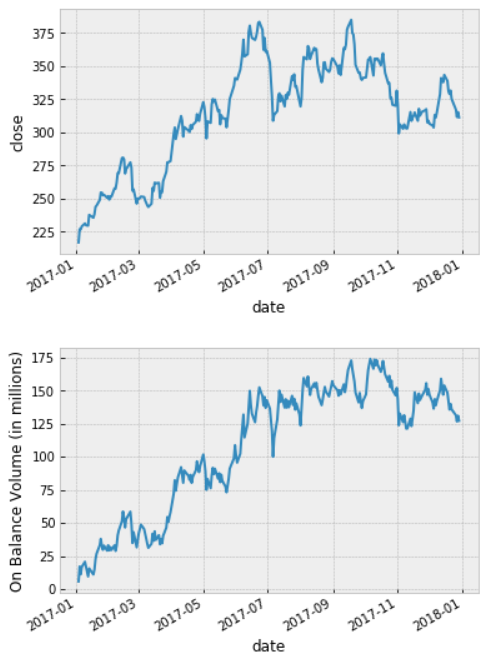You can use a combination of different indicators to create your own strategy. The starter pack of Algorithmic Trading Strategies will help you create quantitative trading strategies using technical indicators which can adapt to live market conditions.

Disclaimer: All investments and trading in the stock market involve risk. Any decisions to place trades in the financial markets, including trading in stock or options or other financial instruments is a personal decision that should only be made after thorough research, including a personal risk and financial assessment and the engagement of professional assistance to the extent you believe necessary. The trading strategies or related information mentioned in this article is for informational purposes only.